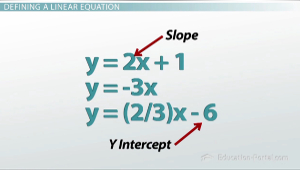# What is a Linear Equation?

An error occurred trying to load this video.

Try refreshing the page, or contact customer support.

Coming up next: Linear Equations: Intercepts, Standard Form and Graphing

### You're on a roll. Keep up the good work!

Replay
Your next lesson will play in 10 seconds
• 0:06 Defining a Linear Equation
• 1:33 Building a Linear Equation
• 2:48 Plotting a Graph
• 5:08 Solving a Linear Equation
• 6:20 Summary

Want to watch this again later?

Timeline
Autoplay
Autoplay
Speed

#### Recommended Lessons and Courses for You

Lesson Transcript
Instructor: Luke Winspur

Luke has taught high school algebra and geometry, college calculus, and has a master's degree in education.

Most cars won't be able to run for more than 250,000 miles, so how much longer will your car live? Linear Equations are the most basic kind of algebraic function and can help you answer questions exactly like this. Learn about what they look like, how they come up in your life and why they are powerful tools.

## Defining a Linear Equation

This lesson is on what a linear equation is. And the answer to that question is essentially a linear equation is any pattern of numbers that is increasing or decreasing by the same amount every step of the way. This means that the only two things that we need to define a linear equation are where the pattern begins and what that pattern moves by. What that leaves us with is the slope-intercept form of the linear equation, y = mx + b, where the m value is the slope, and the b value is the y-intercept.Some examples of linear equations in slope-intercept form: You could have y = 2x + 1; you could have y = -3x; and you could have y = (2/3)x - 6. In each equation, the number in front of the x represents the slope, or the number that it's moving by. The number on the end represents where it begins, or the y-intercept. If there is no number after the x, that implies that the y-intercept is zero.

## Building a Linear Equation

What's the point? Why do I care about a linear equation? Well, linear equations are the most common patterns of numbers we see, and they can be used to describe all sorts of situations you see around you.

One example has to do with the first car I bought. As soon as I turned 16, my parents helped me buy a used car. When I got my car it had 27,000 miles on it, and I've owned it for a while now. Every year, I drive it about 12,000 more miles. What we end up with is a linear equation to represent the situation that looks like y = 12,000x + 27,000, because I drive it 12,000 more miles every year, and that's how much the pattern moves by. And the pattern began at 27,000 miles when I first bought the car. So, after year one, it had 39,000; after year two, it had 51,000; and after year three, it had 63,000. And so on.

## Plotting a Graph

Drawing a graph of this linear equation can help us make a lot of predictions about what this pattern will do in the future, and it can also give us a better sense of the pattern that may not be easy to see just from looking at the numbers. When I want to graph a linear equation, I use the y-intercept, the b value as the beginning value on my graph. So, before I had driven the car a single day, it already had 27,000 miles on it because I got it used. After year one, I'd been driving it along, and after that year I was up to 39,000 miles. I kept driving it and driving it, so after year two I was up to 51,000 miles. So, every time I go over one year, I go up by 12,000 miles.

To unlock this lesson you must be a Study.com Member.

### Register to view this lesson

Are you a student or a teacher?

### Unlock Your Education

#### See for yourself why 30 million people use Study.com

##### Become a Study.com member and start learning now.
Back
What teachers are saying about Study.com

### Earning College Credit

Did you know… We have over 160 college courses that prepare you to earn credit by exam that is accepted by over 1,500 colleges and universities. You can test out of the first two years of college and save thousands off your degree. Anyone can earn credit-by-exam regardless of age or education level.